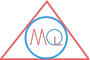MATHS QUERY

# Pythagoras Theorem

Chapter Contents

## Theorem

In a right angled triangle, the square of hypotenuse is equal to the sum of the square of other two sides.

Right angle $$\triangle ABC$$In the above right angle $$\triangle ABC$$, p is perpendicular, b is base and h is hypotenuse.

$$h^2 = b^2 + p^2$$

or $$h = \sqrt{b^2 + p^2}$$

Example

Find the length of hypotenuse of right angle $$\triangle ABC$$, where length of base =3cm and length of perpendicular = 9cm.

Solution:

In $$\triangle ABC$$

b=3cm

p=4cm

$$\because$$, $$\triangle ABC$$ is a right angle triangle          (given)

$$\therefore$$ by applying Pythagoras theorem

$$h = \sqrt{b^2 + p^2}$$

$$h = \sqrt{3^2 + 4^2}$$

$$h = \sqrt{9 + 16}$$

$$h = \sqrt{25}$$

$$\therefore$$ $$h = 5cm$$